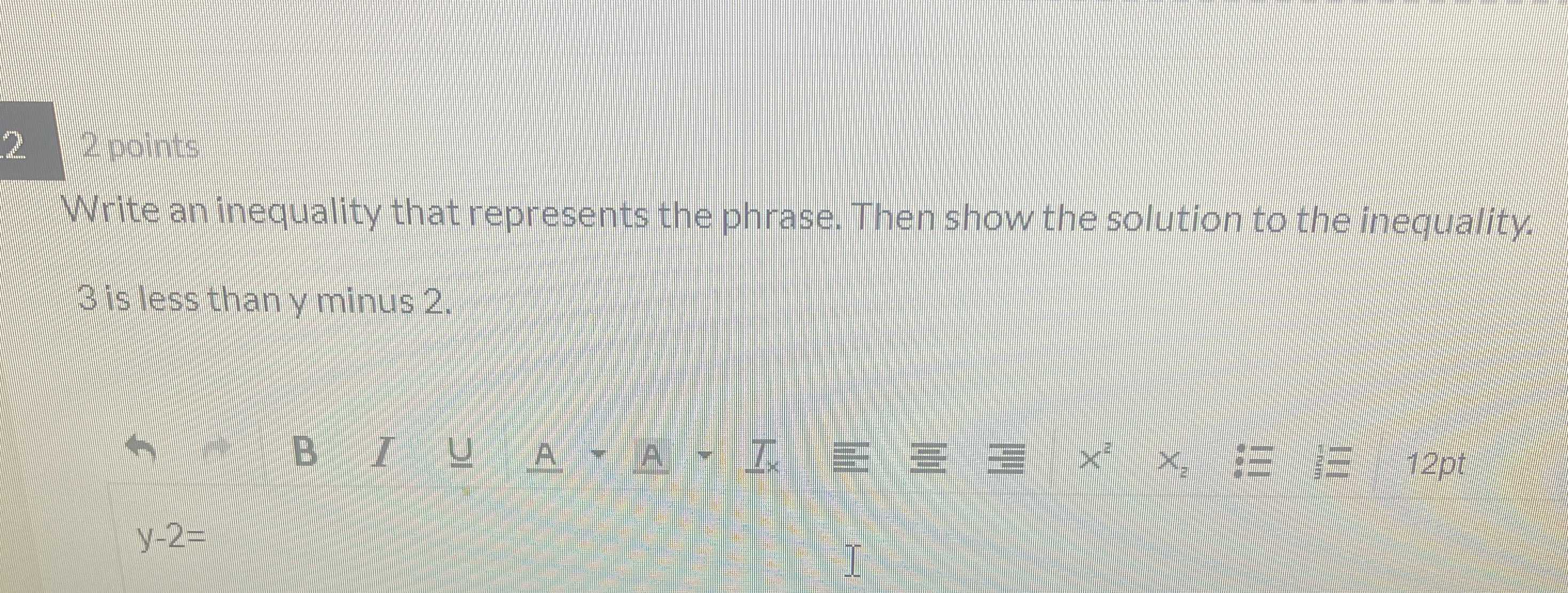### ¿Todavía tienes preguntas de matemáticas?

Pregunte a nuestros tutores expertos
Algebra
Pregunta$$2$$ Write an inequality that represents the phrase. Then show the solution to the inequality.

$$3$$ is less than $$y$$ minus $$2$$ .

$$y - 2 =$$